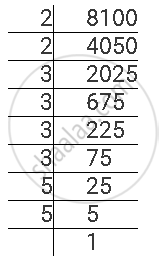Share

# Find the Square Roots of the Given Numbers by the Prime Factorisation Method. 8100 - CBSE Class 8 - Mathematics

ConceptSquare Roots - Finding Square Root Through Prime Factorisation

#### Question

Find the square roots of the given numbers by the Prime Factorisation Method.

8100

#### Solution

8100 can be factorised as follows.8100 = 2 × 2 × 3 × 3 × 3 × 3 × 5 × 5

∴ sqrt(8100) = 2 x 3 x 3 x 5 = 90

Is there an error in this question or solution?

#### APPEARS IN

NCERT Solution for Mathematics Textbook for Class 8 (2018 to Current)
Chapter 6: Squares and Square Roots
Ex. 6.3 | Q: 4.1 | Page no. 102
Solution Find the Square Roots of the Given Numbers by the Prime Factorisation Method. 8100 Concept: Square Roots - Finding Square Root Through Prime Factorisation.
S